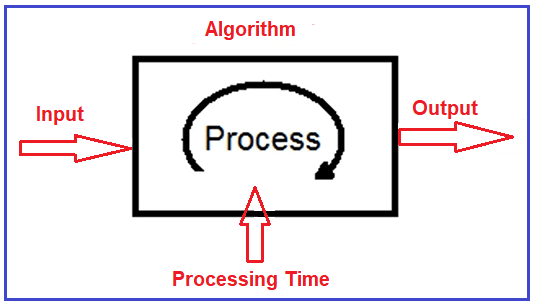Big O Notation in C

# Big O Notation in C

## Big-O Notation in Data Structure

In this article, I am going to discuss Big-O Notation in Data Structure. Please read our previous article where we discussed the Analysis of the Algorithm and why it is important to analyze the algorithm. At the end of this article, you will understand what is Big-O Notation and how to calculate the time complexity of an algorithm using the Big-O Notation in Data Structure.

##### What is Big-O Notation?

Big-O Notation is a symbol or we can say it is a symbolic notation which says that how your algorithm is performed if the input data changes. mostly when the input data increases. What it means. When we talk about the algorithm, algorithms have three pillars. The first one is the Input, the second one is the processing which takes place by the algorithm. And the final one is the output. For better understanding, please have a look at the following diagram. Big-O Notation tells that if your input data increases, then how and in what rate, your algorithm processing time increases. So, it actually tells you the relationship between your processing time and the increase of data.##### Example:

Let us understand the above Big-O Notation definition with an example. Let say you have an algorithm. In that algorithm, let’s assume for 5 records it takes approximately (not exactly) 27 seconds and if we increase the records to 10, it takes 105 seconds and if we further increase the records to 50, it takes approximately 2550 seconds. For better understanding, please have a look at the following image.